Skip to content
Related Articles
Introductory guide to Information Retrieval using KNN and KDTree
• Last Updated : 05 Sep, 2020

## What is Information Retrieval(IR)?

It can be defined as a software program which is used to find material(usual documents) of an unstructured nature(usually text) that satisfies an information need from within large collections(usually stored on computers). It helps users find their required information but does not explicitly return the answers to their questions. It gives information about the existence and location of the documents that might contain the required information.

### Use Cases of Information Retrieval

Information Retrieval can be used in many scenarios, some of these are:

• Web Search
• E-mail Search
• Searching your laptop
• Legal information retrieval etc.

### Process of Information Retrieval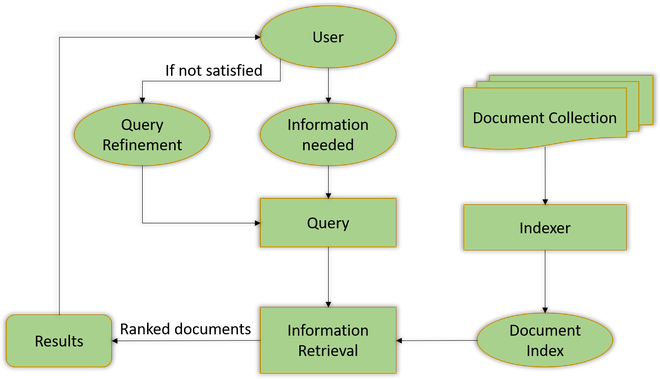To learn more about information retrieval you can refer to this article.

Here we are going to discuss two algorithms which are used in Information Retrieval:

1. K-Nearest Neighbors(KNN)
2. K-Dimensional Tree(KDTree)

## K-Nearest Neighbor (KNN)

It is a supervised machine learning classification algorithm. Classification gives information regarding what group something belongs to, for example, type of tumor, the favourite sport of a person etc. The K in KNN stands for the number of the nearest neighbors that the classifier will use to make its prediction.

We have training data with which we can predict the query data. For the query record which needs to be classified, the KNN algorithm computes the distance between the query record and all of the training data records. Then it looks at the K closest data records in the training data.

How to choose K value?

While choosing K value, keep following these things in mind:

• If K=1, the classes are divided into regions and the query record belongs to a class according to the region it lies in.
• Choose odd values of K for a 2 class problem.
• K must not be a multiple of the number of classes.K not equal to ‘ni’, where n is the number of classes and i = 1, 2, 3….

Distance Metrics: The different distance metrics which can be used are:

• Euclidean Distance
• Manhattan Distance
• Hamming Distance
• Minkowski Distance
• Chebyshev Distance

Let’s take an example to understand in detail how KNN algorithm works. Given below is a small dataset to predict which Pizza outlet a person prefers out of Pizza Hut & Dominos.

The outlet is chosen on the basis of Age of the person and how much Cheese Content does the person like(on the scale of 10).

This data can be visualized graphically as: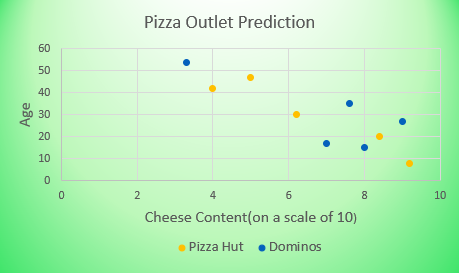Note: If we have discrete data we first have to convert it into numeric data. Ex: Gender is given as Male and Female we can convert it to numeric as 0 and 1.

• Since we have 2 classes — Pizza Hut and Dominos, we’ll take K=3.
• To calculate the distance we are using the Euclidean DistancePrediction: On the basis of the above data we need to find out the result for the query:

• Now find the distance of the record Harry to all the other records.

Given below is the calculation for the distance from Harry to Riya:Similarly, the Distances from all the records are:

From the table we can see that the K(3) closest distances are of Mark, Rachel and Varun and Pizza Outlet they prefer are Pizza Hut, Pizza Hut and Dominos respectively. Hence, we can make the prediction that Harry prefers Pizza Hut.

Advantages of using KNN Algorithm:

• No training phase
• It can learn complex models easily
• It is robust to noisy training data

Disadvantages of using KNN Algorithm:

• Determining the value of parameter K can be difficult as different K values can give different results.
• It is hard to apply on High Dimensional data
• Computation cost is high as for each query it has to go through all the records which take O(N) time, where N is the number of records. If we maintain a priority queue to return the closest K records then the time complexity will be O(log(K)*N).

Due to the high computational cost, we use an algorithm which is time efficient and similar in approach – KDTree Algorithm.

## K-Dimensional Tree (KDTree)

KDTree is a space partitioning data structure for organizing points in K-Dimensional space. It is an improvement over KNN. It is useful for representing data efficiently. In KDTree the data points are organized and partitioned on the basis of some specific conditions.

How the algorithm works:

To understand this let’s take the sample data of Pizza Outlet which we considered in the previous example. Basically we make some axis-aligned cuts and create different regions, keeping the track of points that lie into these regions. Each region is represented by a node in the tree.

• Split the regions at the mean value of the observations.

For the first cut, we’ll find the mean of all the X-coordinates (Cheese Content in this case).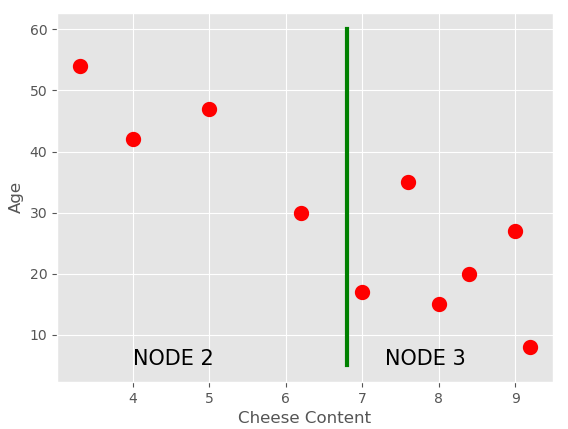Now on both the regions, we’ll calculate the means of Y-coordinates to make the cuts and so on, repeating the steps until the number of points in each region is less than a given number. You can choose any number less than the number of records in the dataset otherwise we’ll have only 1 region. The complete tree structure for this will be: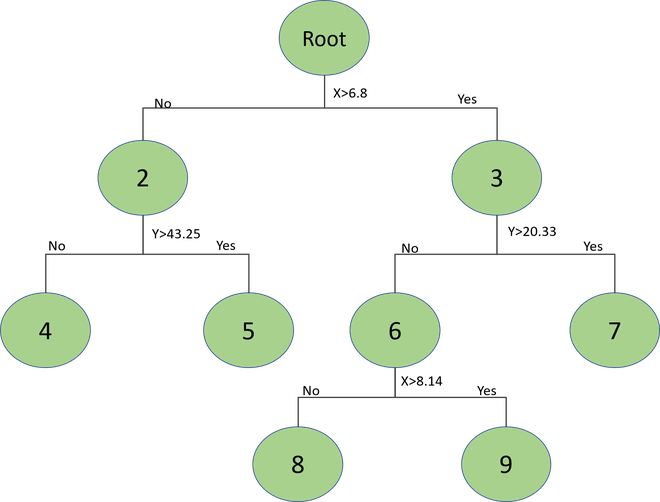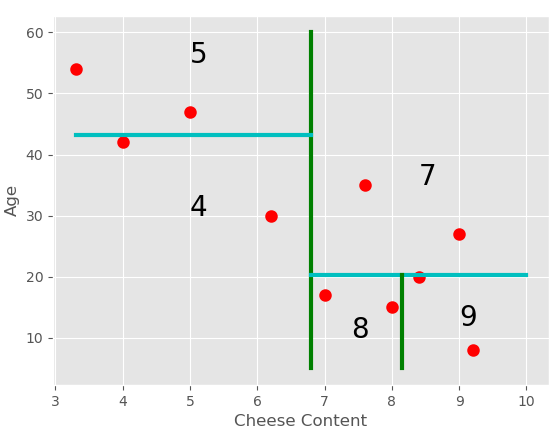Here there are less than 3 points in each region.

Now if a new query point comes, and we need to find out in which region will the point be, we can traverse the tree. In this case, the query point(chosen randomly) lies in the 4th region.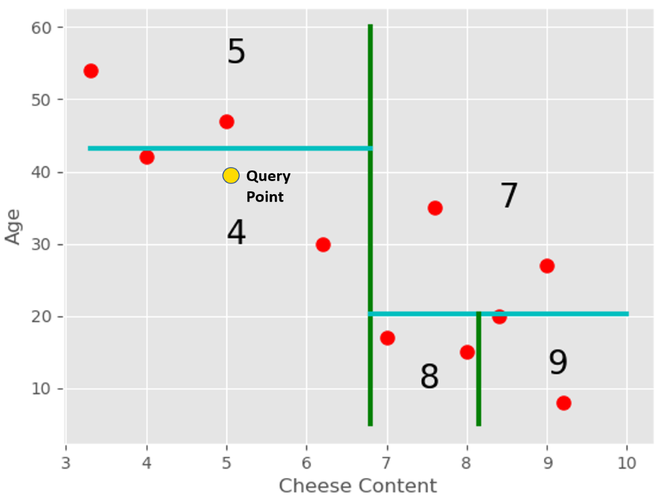We can find it’s the nearest neighbour in this region.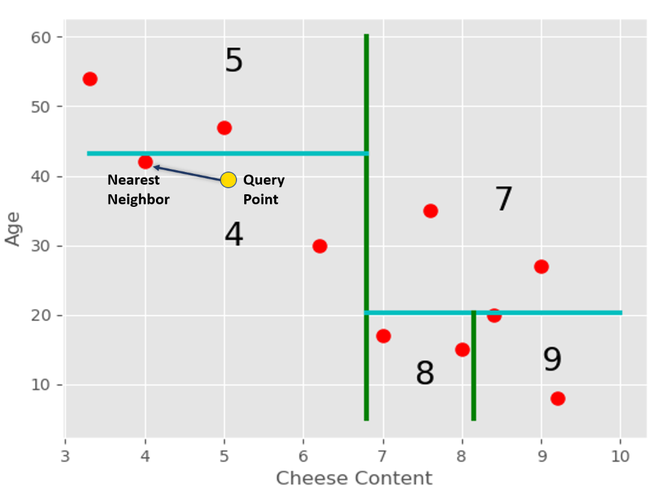But this might not be the actual the nearest neighbour for this query in the entire dataset. Hence we traverse back to node 2 and then check the remaining subtree for this node.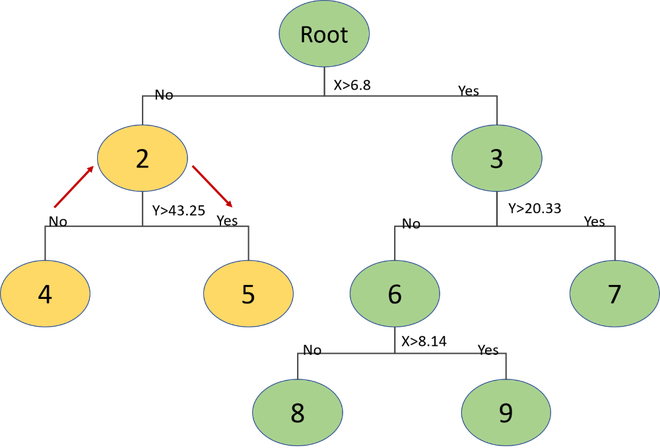We get the tightest box for node 5 which contains all the points in this region. After that, we check if the distance of this box is closer to the query point than the current nearest neighbour or not.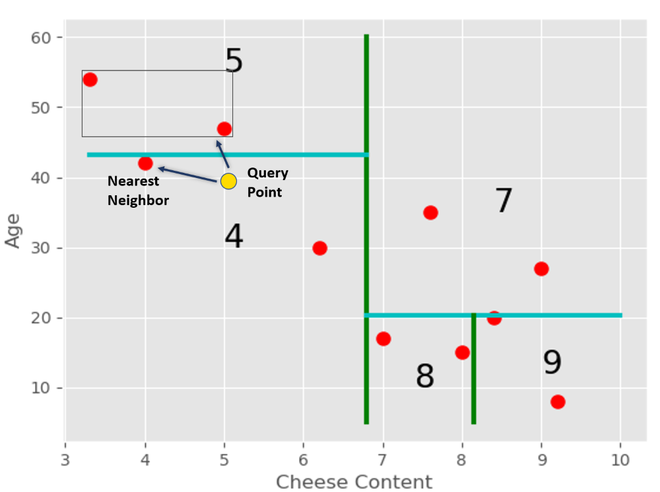In this case, the distance of the box is smaller. Hence, there is a point in region point which is closer to the query point than the current the nearest neighbour. We find that point, and then we again traverse back the tree to node 3 and check the same.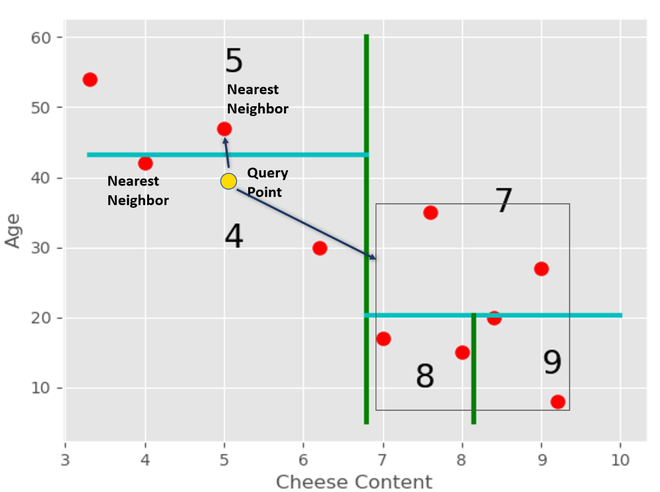Now the distance is greater than the distance from the new nearest neighbour. Hence, we stop here, and we do not need to search for this sub-tree. We can prune this part of the tree.

Note: A branch of the tree is eliminated only when K points have been found and the branch cannot have points closer than any of the K current bests.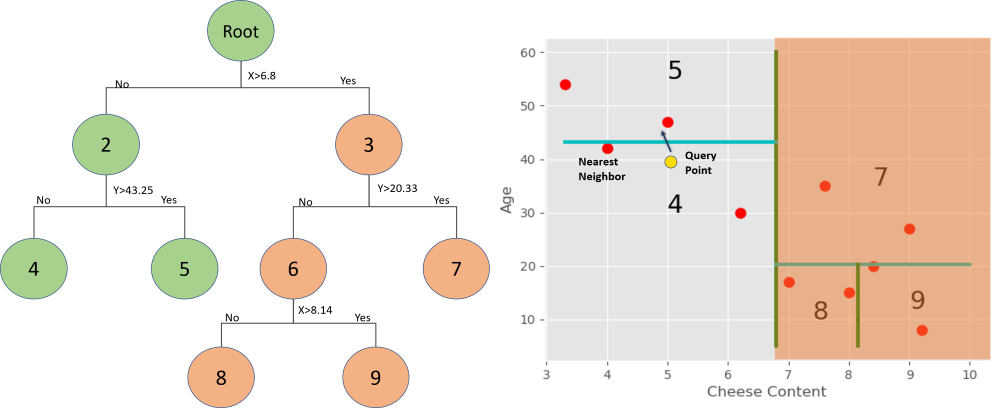KDtree Implementation:

We will be performing Document Retrieval which is the most widely used use case for Information Retrieval. For this, we have made a sample dataset of articles available on the internet on famous celebrities. On entering the name you get the names of the celebrities similar to the given name. Here the K value is taken as 3. We will get three nearest neighbors of the document name entered.

You can get the dataset from here.

Code:

 #import the libraries requiredimport numpy as npimport pandas as pdimport nltkfrom sklearn.feature_extraction.text import CountVectorizer, TfidfTransformerfrom sklearn.neighbors import KDTree  #Reading the datasetperson = pd.read_csv('famous_people.csv')  #Printing first five rows of the datasetprint(person.head())

Output: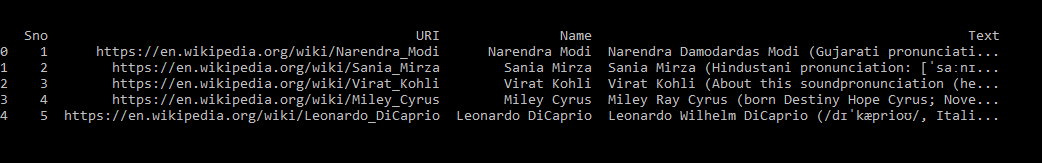Code:

 #Counting the frequency of occurance of each wordcount_vector = CountVectorizer()train_counts = count_vector.fit_transform(person.Text)  #Using tf-idf to reduce the weight of common wordstfidf_transform = TfidfTransformer()train_tfidf = tfidf_transform.fit_transform(train_counts)a = np.array(train_tfidf.toarray())  #obtaining the KDTreekdtree = KDTree(a ,leaf_size=3)  #take the name of the personality as inputperson_name=input("Enter the name of the Person:- ")  #Using KDTree to get K articles similar to the given nameperson['tfidf']=list(train_tfidf.toarray())distance, idx = kdtree.query(person['tfidf'][person['Name']== person_name].tolist(), k=3)for i, value in list(enumerate(idx)):    print("Name : {}".format(person['Name'][value]))    print("Distance : {}".format(distance[i]))    print("URI : {}".format(person['URI'][value]))

Output: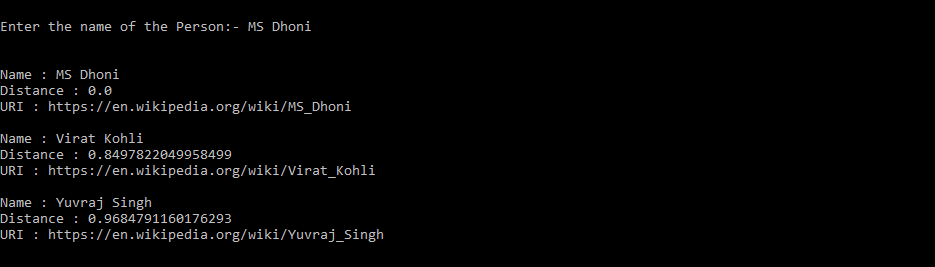We get MS Dhoni, Virat Kohli and Yuvraj Singh as the 3 nearest neighbors for MS Dhoni.

### Advantages of using KDTree

• At each level of the tree, KDTree divides the range of the domain in half.Hence they are useful for performing range searches.
• It is an improvement of KNN as discussed earlier.
• The complexity lies in between O(log N) to O(N) where N is the number of nodes in the tree.

### Disadvantages of using KDTree

• Degradation of performance when high dimensional data is used.The algorithm will need to visit many more branches.If the dimensionality of dataset is K then the number of nodes N>>(2^K).
• If the query point if far from all the points in the dataset then we might have to traverse the whole tree to find the nearest neighbors.

For any queries do leave a comment below.My Personal Notes arrow_drop_up